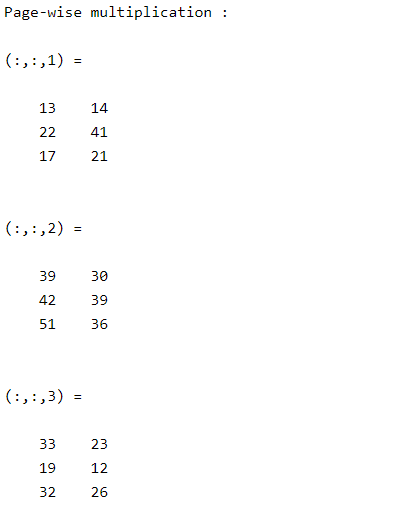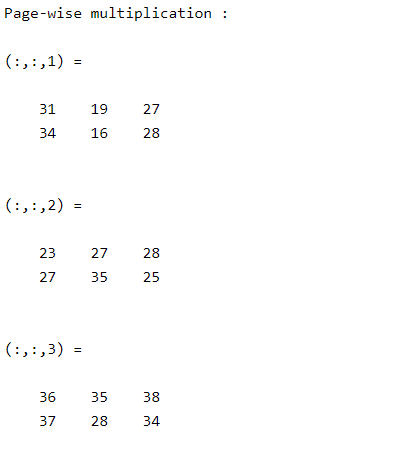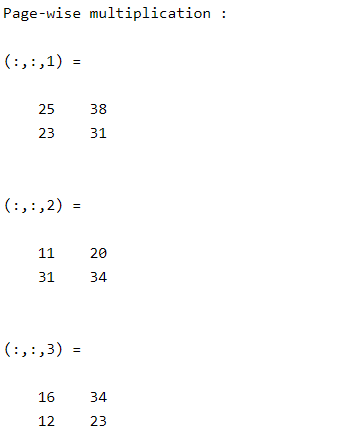# Page-wise matrix multiplication in MATLAB

• Last Updated : 04 Jul, 2021

Page-wise matrix multiplication is multiplying two N-D arrays along each dimension or page of two arrays. Matlab allows users to calculate page-wise multiplication using pagemtimes().

Different syntax of pagemtimes() method are:

• Z = pagemtimes(X,Y)
• Z = pagemtimes(X,transpX,Y,transpY)

### Z = pagemtimes(X,Y)

• It returns the page-wise multiplication of N-D arrays X and Y. Each page product is given by Z(: , : , i) = X(: , : , i)*Y(: , : , i).
• If X and Y are N-D matrices then the first 2 dimensions of X and Y should be compatible with matrix multiplication.

Example:

## Matlab

 `% Two 3-D arrays of sizes 3*3*3 and 3*2*3``X = randi([1 6],3,3,3);``Y = randi([1 6],3,2,3);`` ` `% Page-wise multiplication``M = pagemtimes(X,Y);``disp(``"Page-wise multiplication :"``);``disp(M);`

Output :If X is a matrix and Y N-D array, then  Z(: , : , i) = X*Y(: , : , I). Dimensions of X should be compatible with the first 2 dimensions of Y.

Example:

## Matlab

 `% X is a matrix of 2*3``% y is 3-D array of 3*3*3``X = randi([1 6],2,3);``Y = randi([1 6],3,3,3);`` ` `% Page-wise multiplication``M = pagemtimes(X,Y);``disp(``"Page-wise multiplication :"``);``disp(M);`

Output :### Z = pagemtimes(X, transpX, Y, transpY)

• It returns the page-wise multiplication of X and Y by considering transpositions of X and Y as transpX and transpY.
• If transp = ‘none’, then it doesn’t change the transposition of the array
• If transp = ‘transpose’, then it transposes every page of the array.
• If transp = ‘ctranspose’, then it applies complex conjugate transpose on every page of the array.

Example:

## Matlab

 `% X is a 3-D array of 3*2*3``% y is 3-D array of 3*3*3``X = randi([1 6],3,2,3);``Y = randi([1 6],3,2,3);`` ` `% Page-wise multiplication as X'*Y``M = pagemtimes(X,``'transpose'``,Y,``'none'``);``disp(``"Page-wise multiplication :"``);``disp(M);`

Output :My Personal Notes arrow_drop_up